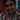# Defensive coding with guard assertions in Javascript

October 06, 2019 - 3 min read

Let’s take a look at a function that took a score between 1 and 100 and returned a rating out of 5.

``````function rating(score) {
return Math.ceil(score / 20);
}``````

At first glance, this function looks simple, it has made a lot of assumption about its input argument. The obvious assumption is that the score is between 1 and 100. The not so obvious assumption is that it is a number. As Javascript is an un-typed language, any client calling this function can pass in the score as `"21"` or even `"foo"`.

What should the return value of this function be if the input `score` is a string? Should it return `NaN`? throw an exception? or return `-1`?

It may be that in the context where this function is being used, it will never get into the erroneous state. For example, `rating(toPercentage(score, 1, 10))`. Let’s assume that `toPercentage` is returning a percentage given a score between 1 and 10. So, in this context, we can see that the function `rating` is actually used to convert a score from 1 to 10 to 1 to 5. Perhaps, `toPercentage` handles the case when the initial score variable is not a number between 1, to 10 (by capping the value), or the case when the score is a string returning 0 etc. We don’t know without looking in the source code of the function. The `toPercentage` function could’ve been provided by an `npm` package maintained by a different team. Who knows, they might start returning you a percentage as a string in later versions. This has happened to me in a project where the score was returned by an API call. And went from being a `number` to being a `string`.

How would you know if the `score` variable starts behaving differently to when you wrote the `rating` method? How can we catch this in production and make fixing it easier.

How about writting these assumptions down?

``````function rating(score) {
console.assert(
score !== null || score !== undefined,
"expected score to be not null or undefined"
);
console.assert(Number.isFinite(score), "expected score to be a valid number");
console.assert(
score >= 1 && score <= 100,
"expected score to be between 1 and 100"
);
return Math.ceil(score / 20);
}``````

This is starting to look a lot like defensive programming. A method should always validate its input. Refactoring by Martin Fowler has documented this as Introduce Assertions. This seems a bit verbose and the lines of code have gone from 1 to 4.

``````function rating(score) {
assert.isBetween(1, 100, "score", score);
return Math.ceil(score / 20);
}``````

In this variation, `assert.isBetween` function can handle the score being `undefined` or `null`. Also ensuring the type being a number and finally in the acceptable range.

``````function isBetween(lower, upper, name, value) {
console.assert(
score !== null || score !== undefined,
`expected \${name} to be not null or undefined`
);
console.assert(
Number.isFinite(value),
`expected \${name} to be a valid number, but was \${value}.`
);
console.assert(
value >= lower && value <= upper,
`expected \${name} to be between \${lower} and \${upper}.`
);
return true;
}``````

## Conclusion

Even though defensive coding can get verbose, the benefits of doing so can help diagnose errors. I have seen this kind of coding in C#, I have not run into it in Javascript codebases. Given the untyped nature of the language, it seems logical to start writing code this way. The `isBetween` assertion function is verbose to make it easier to understand. Using a library such as ramdajs, can help compose the `isBetween` function, shortening it.Written by Harish H. Babu who lives and works in Cardiff engineering software things. You should follow him on Twitter